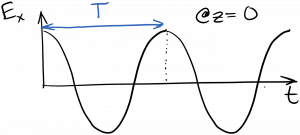Laser Photonics Time-harmonic waves

# Time-harmonic waves

We saw that the solutions to the scalar wave equation were of the form$\displaystyle E_x = f(z \pm v_p t)$

where$f$ was any twice-differentiable function.  I’m going to start using sinusoidal  or harmonic functions for$f$ – functions like cosine, sine, and complex exponentials.  Let’s start with cosine.  We should certainly be allowed to replace$f$ with a cosine, as it is twice differentiable.  If we do so, we have$\displaystyle E_x = A \cos {(k(z - v_p t))}$

I decided to go with the minus sign for the forward-propagating wave (wave traveling in the$+z$ direction), and I slipped in a few new constants:$A$ out front, which is just the amplitude of the cosine wave; and a$k$ inside the cosine, which I’ll discuss below.  Neither of these constants changes the fact that this harmonic wave is a solution to the wave equation, because it’s still twice-differentiable, and it still has the necessary$(z - v_p t)$ grouping inside.  I’m going to rewrite the harmonic wave a bit without really changing it.  First I’ll bring the$k$ inside the parentheses:$\displaystyle E_x = A \cos {(kz - k v_p t)}$

The quantity in front of$t$ in a harmonic function is just the angular frequency$\omega$, so we can rewrite this as$\displaystyle E_x = A \cos {(kz - \omega t)}$

where we must have$\displaystyle \omega = k v_p$

You probably have some intuitive feel for the angular frequency$\omega = 2 \pi \nu$, where$nu$ is the ordinary frequency, often given in Hertz ($Hz$).  For example, as$\omega$ gets bigger, the harmonic function is oscillating faster in time.  The period of oscillation – the time it takes for the oscillation to undergo one complete cycle – is just$\displaystyle T = \frac{1}{\nu} = \frac{2 \pi}{\omega}$Slightly newer is$k$, which is called the wavenumber.  Looking at our harmonic wave, notice that$k$ sits in front of$z$, just as$\omega$ sits in front of$t$.  In fact, the wavenumber$k$ plays the same role as$\omega$ does, except that it applies to the wave’s oscillation in space, rather than time.  So, as$k$ gets bigger, the harmonic wave is oscillating faster in space.  We can think of the wavenumber$k$ as being an angular spatial frequency.

The wavelength$\lambda$ is the spatial distance between peaks of the wave; i.e. it’s the distance over which the oscillation undergoes one complete cycle.  It’s analogous to the period, but applied to space, rather than time.  Similar to how the period$T = 2 \pi / \omega$, the wavelength$\displaystyle \lambda = 2 \pi / k$.We can do a little manipulation here to come up with a very famous equation.  We already know that$\displaystyle \omega = k v_p$

and we know$\omega = 2 \pi \nu$, and$k = 2 \pi / \lambda$.  In the next section we will see that the phase velocity$v_p = c / n$.  Putting all of this together and rearranging things,$\displaystyle \lambda \nu = \frac{c}{n}$

In words, wavelength times frequency equals the speed of light divided by the refractive index.  This holds for all electromagnetic waves, including light.

Below are some animations to illustrate the connections between$\lambda$,$v_p$, and$\omega$.

Animation showing a wave with amplitude A = 1,$\lambda = 1$, and$v_p = 1$:

waveanimation111

Animation showing a wave with amplitude A = 2,$\lambda = 1$, and$v_p = 1$:

waveanimation211

Animation showing a wave with amplitude A = 1,$\lambda = 0.5$, and$v_p = 1$:

waveanimation1half1

(Notice how the frequency$\omega$ has increased, because the faster spatial oscillations translate into faster time oscillations when the wave passes at a fixed speed.)

Animation showing a wave with amplitude A = 1,$\lambda = 1$, and$v_p = 2$:

waveanimation112

(Notice how the frequency$\omega$ has increased, even though the spatial frequency$k$ have not, because the spatial oscillations are passing by faster.)